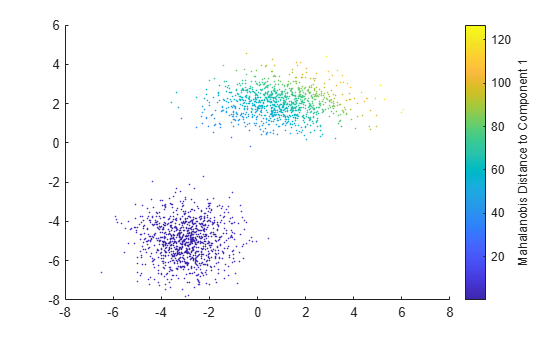Documentation

# mahal

Mahalanobis distance to Gaussian mixture component

## Syntax

``d2 = mahal(gm,X)``

## Description

example

````d2 = mahal(gm,X)` returns the squared Mahalanobis distance of each observation in `X` to each Gaussian mixture component in `gm`.```

## Examples

collapse all

Generate random variates that follow a mixture of two bivariate Gaussian distributions by using the `mvnrnd` function. Fit a Gaussian mixture model (GMM) to the generated data by using the `fitgmdist` function, and then compute Mahalanobis distances between the generated data and the mixture components of the fitted GMM.

Define the distribution parameters (means and covariances) of two bivariate Gaussian mixture components.

```rng('default') % For reproducibility mu1 = [1 2]; % Mean of the 1st component sigma1 = [2 0; 0 .5]; % Covariance of the 1st component mu2 = [-3 -5]; % Mean of the 2nd component sigma2 = [1 0; 0 1]; % Covariance of the 2nd component```

Generate an equal number of random variates from each component, and combine the two sets of random variates.

```r1 = mvnrnd(mu1,sigma1,1000); r2 = mvnrnd(mu2,sigma2,1000); X = [r1; r2];```

The combined data set `X` contains random variates following a mixture of two bivariate Gaussian distributions.

Fit a two-component GMM to `X`.

`gm = fitgmdist(X,2)`
```gm = Gaussian mixture distribution with 2 components in 2 dimensions Component 1: Mixing proportion: 0.500000 Mean: -2.9617 -4.9727 Component 2: Mixing proportion: 0.500000 Mean: 0.9539 2.0261 ```

`fitgmdist` fits a GMM to `X` using two mixture components. The means of `Component` `1` and `Component` `2` are `[-2.9617,-4.9727]` and `[0.9539,2.0261]`, which are close to `mu2` and `mu1`, respectively.

Compute the Mahalanobis distance of each point in `X` to each component of `gm`.

`d2 = mahal(gm,X);`

Plot `X` by using `scatter` and use marker color to visualize the Mahalanobis distance to `Component` `1`.

```scatter(X(:,1),X(:,2),10,d2(:,1),'.') % Scatter plot with points of size 10 c = colorbar; ylabel(c,'Mahalanobis Distance to Component 1')```## Input Arguments

collapse all

Gaussian mixture distribution, also called Gaussian mixture model (GMM), specified as a `gmdistribution` object.

You can create a `gmdistribution` object using `gmdistribution` or `fitgmdist`. Use the `gmdistribution` function to create a `gmdistribution` object by specifying the distribution parameters. Use the `fitgmdist` function to fit a `gmdistribution` model to data given a fixed number of components.

Data, specified as an n-by-m numeric matrix, where n is the number of observations and m is the number of variables in each observation.

If a row of `X` contains `NaNs`, then `mahal` excludes the row from the computation. The corresponding value in `d2` is `NaN`.

Data Types: `single` | `double`

## Output Arguments

collapse all

Squared Mahalanobis distance of each observation in `X` to each Gaussian mixture component in `gm`, returned as an n-by-k numeric matrix, where n is the number of observations in `X` and k is the number of mixture components in `gm`.

`d2(i,j)` is the squared distance of observation `i` to the `j`th Gaussian mixture component.

collapse all

### Mahalanobis Distance

The Mahalanobis distance is a measure between a sample point and a distribution.

The Mahalanobis distance from a vector x to a distribution with mean μ and covariance Σ is

`$d=\sqrt{\left(x-\mu \right){\sum }^{-1}\left(x-\mu \right)\text{'}}.$`

This distance represents how far x is from the mean in number of standard deviations.

`mahal` returns the squared Mahalanobis distance d2 from an observation in `X` to a mixture component in `gm`.